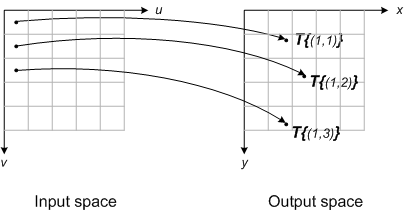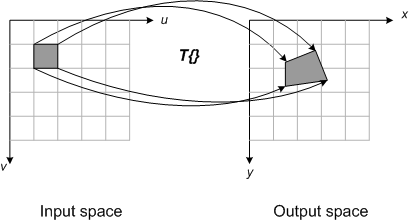Spatial transformations: Forward mapping

I've written previously about defining a spatial transform as a function, (x,y) = T{(u,v)}, that maps points from one space (input space) to another (output space). Given such a function, how do you go about spatially transforming an image?

The most obvious procedure is called forward mapping. It works like this:

Consider each input image pixel in turn. For each input image pixel:

• Determine its location in input space, (uk,vk).
• Map that location to output space using (xk,yk) = T{(uk,vk)}.
• Figure out which output pixel contains the location (xk,yk).
• Copy the input pixel value to that output pixel.
This diagram illustrates the procedure:Forward mapping has two main disadvantages as a computational procedure: gaps and overlaps. Depending on specific spatial transform function, you may have some output pixels that did not receive any input image pixels; these are the gaps. You may also have some output pixels that received more than one input image pixel; these are the overlaps. In both cases, it is challenging to figure out a reasonable way to set those output pixels.

One way to overcome these problems is to map pixel rectangles in input space to output space quadrilaterals, as shown here:With this procedure, the input pixel value is allocated to different output pixels depending on the relative fractional coverage of the quadrilateral. Although this procedure can produce good results, it is complicated to implement and it takes a long time to compute.

Because of the problems with forward mapping, many (most?) image spatial transform implementations use a different technique, called inverse mapping. I'll describe that in a future post.

|